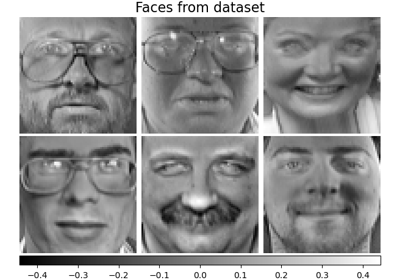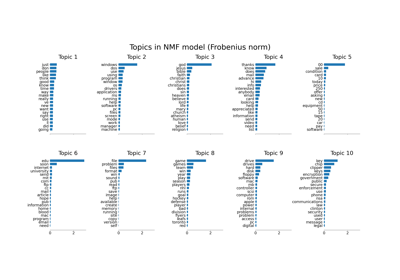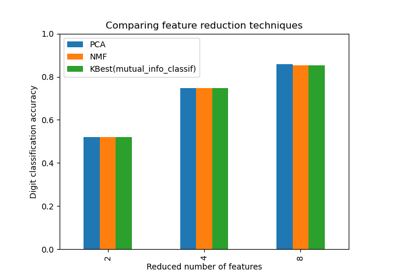# sklearn.decomposition.NMF¶

class sklearn.decomposition.NMF(n_components=None, *, init=None, solver='cd', beta_loss='frobenius', tol=0.0001, max_iter=200, random_state=None, alpha_W=0.0, alpha_H='same', l1_ratio=0.0, verbose=0, shuffle=False)[source]

Non-Negative Matrix Factorization (NMF).

Find two non-negative matrices, i.e. matrices with all non-negative elements, (W, H) whose product approximates the non-negative matrix X. This factorization can be used for example for dimensionality reduction, source separation or topic extraction.

The objective function is:

\begin{align}\begin{aligned}L(W, H) &= 0.5 * ||X - WH||_{loss}^2\\&+ alpha\_W * l1\_ratio * n\_features * ||vec(W)||_1\\&+ alpha\_H * l1\_ratio * n\_samples * ||vec(H)||_1\\&+ 0.5 * alpha\_W * (1 - l1\_ratio) * n\_features * ||W||_{Fro}^2\\&+ 0.5 * alpha\_H * (1 - l1\_ratio) * n\_samples * ||H||_{Fro}^2\end{aligned}\end{align}

Where:

$$||A||_{Fro}^2 = \sum_{i,j} A_{ij}^2$$ (Frobenius norm)

$$||vec(A)||_1 = \sum_{i,j} abs(A_{ij})$$ (Elementwise L1 norm)

The generic norm $$||X - WH||_{loss}$$ may represent the Frobenius norm or another supported beta-divergence loss. The choice between options is controlled by the beta_loss parameter.

The regularization terms are scaled by n_features for W and by n_samples for H to keep their impact balanced with respect to one another and to the data fit term as independent as possible of the size n_samples of the training set.

The objective function is minimized with an alternating minimization of W and H.

Note that the transformed data is named W and the components matrix is named H. In the NMF literature, the naming convention is usually the opposite since the data matrix X is transposed.

Read more in the User Guide.

Parameters:
n_componentsint, default=None

Number of components, if n_components is not set all features are kept.

init{‘random’, ‘nndsvd’, ‘nndsvda’, ‘nndsvdar’, ‘custom’}, default=None

Method used to initialize the procedure. Valid options:

• None: ‘nndsvda’ if n_components <= min(n_samples, n_features), otherwise random.

• 'random': non-negative random matrices, scaled with: sqrt(X.mean() / n_components)

• 'nndsvd': Nonnegative Double Singular Value Decomposition (NNDSVD) initialization (better for sparseness)

• 'nndsvda': NNDSVD with zeros filled with the average of X (better when sparsity is not desired)

• 'nndsvdar' NNDSVD with zeros filled with small random values (generally faster, less accurate alternative to NNDSVDa for when sparsity is not desired)

• 'custom': Use custom matrices W and H which must both be provided.

Changed in version 1.1: When init=None and n_components is less than n_samples and n_features defaults to nndsvda instead of nndsvd.

solver{‘cd’, ‘mu’}, default=’cd’

Numerical solver to use:

• ‘cd’ is a Coordinate Descent solver.

• ‘mu’ is a Multiplicative Update solver.

New in version 0.17: Coordinate Descent solver.

New in version 0.19: Multiplicative Update solver.

beta_lossfloat or {‘frobenius’, ‘kullback-leibler’, ‘itakura-saito’}, default=’frobenius’

Beta divergence to be minimized, measuring the distance between X and the dot product WH. Note that values different from ‘frobenius’ (or 2) and ‘kullback-leibler’ (or 1) lead to significantly slower fits. Note that for beta_loss <= 0 (or ‘itakura-saito’), the input matrix X cannot contain zeros. Used only in ‘mu’ solver.

New in version 0.19.

tolfloat, default=1e-4

Tolerance of the stopping condition.

max_iterint, default=200

Maximum number of iterations before timing out.

random_stateint, RandomState instance or None, default=None

Used for initialisation (when init == ‘nndsvdar’ or ‘random’), and in Coordinate Descent. Pass an int for reproducible results across multiple function calls. See Glossary.

alpha_Wfloat, default=0.0

Constant that multiplies the regularization terms of W. Set it to zero (default) to have no regularization on W.

New in version 1.0.

alpha_Hfloat or “same”, default=”same”

Constant that multiplies the regularization terms of H. Set it to zero to have no regularization on H. If “same” (default), it takes the same value as alpha_W.

New in version 1.0.

l1_ratiofloat, default=0.0

The regularization mixing parameter, with 0 <= l1_ratio <= 1. For l1_ratio = 0 the penalty is an elementwise L2 penalty (aka Frobenius Norm). For l1_ratio = 1 it is an elementwise L1 penalty. For 0 < l1_ratio < 1, the penalty is a combination of L1 and L2.

New in version 0.17: Regularization parameter l1_ratio used in the Coordinate Descent solver.

verboseint, default=0

Whether to be verbose.

shufflebool, default=False

If true, randomize the order of coordinates in the CD solver.

New in version 0.17: shuffle parameter used in the Coordinate Descent solver.

Attributes:
components_ndarray of shape (n_components, n_features)

Factorization matrix, sometimes called ‘dictionary’.

n_components_int

The number of components. It is same as the n_components parameter if it was given. Otherwise, it will be same as the number of features.

reconstruction_err_float

Frobenius norm of the matrix difference, or beta-divergence, between the training data X and the reconstructed data WH from the fitted model.

n_iter_int

Actual number of iterations.

n_features_in_int

Number of features seen during fit.

New in version 0.24.

feature_names_in_ndarray of shape (n_features_in_,)

Names of features seen during fit. Defined only when X has feature names that are all strings.

New in version 1.0.

DictionaryLearning

Find a dictionary that sparsely encodes data.

MiniBatchSparsePCA

Mini-batch Sparse Principal Components Analysis.

PCA

Principal component analysis.

SparseCoder

Find a sparse representation of data from a fixed, precomputed dictionary.

SparsePCA

Sparse Principal Components Analysis.

TruncatedSVD

Dimensionality reduction using truncated SVD.

References



“Fast local algorithms for large scale nonnegative matrix and tensor factorizations” Cichocki, Andrzej, and P. H. A. N. Anh-Huy. IEICE transactions on fundamentals of electronics, communications and computer sciences 92.3: 708-721, 2009.



“Algorithms for nonnegative matrix factorization with the beta-divergence” Fevotte, C., & Idier, J. (2011). Neural Computation, 23(9).

Examples

>>> import numpy as np
>>> X = np.array([[1, 1], [2, 1], [3, 1.2], [4, 1], [5, 0.8], [6, 1]])
>>> from sklearn.decomposition import NMF
>>> model = NMF(n_components=2, init='random', random_state=0)
>>> W = model.fit_transform(X)
>>> H = model.components_


Methods

 fit(X[, y]) Learn a NMF model for the data X. fit_transform(X[, y, W, H]) Learn a NMF model for the data X and returns the transformed data. get_feature_names_out([input_features]) Get output feature names for transformation. get_params([deep]) Get parameters for this estimator. Transform data back to its original space. set_output(*[, transform]) Set output container. set_params(**params) Set the parameters of this estimator. Transform the data X according to the fitted NMF model.
fit(X, y=None, **params)[source]

Learn a NMF model for the data X.

Parameters:
X{array-like, sparse matrix} of shape (n_samples, n_features)

Training vector, where n_samples is the number of samples and n_features is the number of features.

yIgnored

Not used, present for API consistency by convention.

**paramskwargs

Parameters (keyword arguments) and values passed to the fit_transform instance.

Returns:
selfobject

Returns the instance itself.

fit_transform(X, y=None, W=None, H=None)[source]

Learn a NMF model for the data X and returns the transformed data.

This is more efficient than calling fit followed by transform.

Parameters:
X{array-like, sparse matrix} of shape (n_samples, n_features)

Training vector, where n_samples is the number of samples and n_features is the number of features.

yIgnored

Not used, present for API consistency by convention.

Warray-like of shape (n_samples, n_components), default=None

If init='custom', it is used as initial guess for the solution. If None, uses the initialisation method specified in init.

Harray-like of shape (n_components, n_features), default=None

If init='custom', it is used as initial guess for the solution. If None, uses the initialisation method specified in init.

Returns:
Wndarray of shape (n_samples, n_components)

Transformed data.

get_feature_names_out(input_features=None)[source]

Get output feature names for transformation.

The feature names out will prefixed by the lowercased class name. For example, if the transformer outputs 3 features, then the feature names out are: ["class_name0", "class_name1", "class_name2"].

Parameters:
input_featuresarray-like of str or None, default=None

Only used to validate feature names with the names seen in fit.

Returns:
feature_names_outndarray of str objects

Transformed feature names.

get_params(deep=True)[source]

Get parameters for this estimator.

Parameters:
deepbool, default=True

If True, will return the parameters for this estimator and contained subobjects that are estimators.

Returns:
paramsdict

Parameter names mapped to their values.

inverse_transform(W)[source]

Transform data back to its original space.

New in version 0.18.

Parameters:
W{ndarray, sparse matrix} of shape (n_samples, n_components)

Transformed data matrix.

Returns:
X{ndarray, sparse matrix} of shape (n_samples, n_features)

Returns a data matrix of the original shape.

set_output(*, transform=None)[source]

Set output container.

See Introducing the set_output API for an example on how to use the API.

Parameters:
transform{“default”, “pandas”}, default=None

Configure output of transform and fit_transform.

• "default": Default output format of a transformer

• "pandas": DataFrame output

• None: Transform configuration is unchanged

Returns:
selfestimator instance

Estimator instance.

set_params(**params)[source]

Set the parameters of this estimator.

The method works on simple estimators as well as on nested objects (such as Pipeline). The latter have parameters of the form <component>__<parameter> so that it’s possible to update each component of a nested object.

Parameters:
**paramsdict

Estimator parameters.

Returns:
selfestimator instance

Estimator instance.

transform(X)[source]

Transform the data X according to the fitted NMF model.

Parameters:
X{array-like, sparse matrix} of shape (n_samples, n_features)

Training vector, where n_samples is the number of samples and n_features is the number of features.

Returns:
Wndarray of shape (n_samples, n_components)

Transformed data.

## Examples using sklearn.decomposition.NMF¶Faces dataset decompositions

Faces dataset decompositionsTopic extraction with Non-negative Matrix Factorization and Latent Dirichlet Allocation

Topic extraction with Non-negative Matrix Factorization and Latent Dirichlet AllocationSelecting dimensionality reduction with Pipeline and GridSearchCV

Selecting dimensionality reduction with Pipeline and GridSearchCV